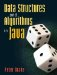# The Queue Interface

### Projects

 4.2 Java uses infix notation for arithmetic. In infix notation, the operator (+, -, *, or /) appears in between the operands. For example: ```2 + 2 3 / 5 ```   An alternative is postfix notation, where the operator appears at the end. The expressions above would be written as ```2 2 + 3 5 / ```   The advantage of postfix notation is that complicated expressions do not require parentheses. For example, using infix notation, we need parentheses for the expression: ```(5 - 2) * 4 ```   The value of this expression is 12. If we leave out the parentheses, this becomes ```5 - 2 * 4 ```   which is -3. Using postfix notation, the first expression is ```5 2 - 4 * ```   but the second one is ```5 2 4 * - ```   No parentheses, no ambiguity! The behavior of a postfix calculator can be understood in terms of a stack. The stack is initially empty. Whenever a number is entered, it is pushed onto the stack. Whenever an operator is entered, the top two numbers on the stack are popped and combined with the appropriate operator. The result is pushed onto the stack. Write a postfix calculator, in the form of a class Calc. Figure 4-39 shows the calculator at work.

Figure 4-39. The postfix calculator (Project 4.20) at work.

 ``` 1 : 2 2 2.0: 2 3 2.0: + 4 4.0: 5 5 5.0: * 6 20.0: 7 7 7.0: 2 8 2.0: / 9 3.5: - 10 16.5: quit ```
 Notice that the calculator prints the top item on the stack (if any) to the left of the prompt. Your calculator should support the operators +, *, -, and /, as well as the command quit. Use doubles, not ints. Make sure that, if the user enters 5, 3, and -, he gets 2, not 2. Hint: Read in a String with INPUT.nextLine(). After you are sure that the String is not one of the commands mentioned, you can convert it into a double with the method Double.parseDouble().Data Structures and Algorithms in Java
ISBN: 0131469142
EAN: 2147483647
Year: 2004
Pages: 216
Authors: Peter Drake